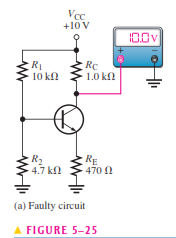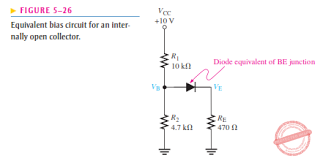# Floyd Self-test in Transistor Bias Circuits

(Last Updated On: December 5, 2019)This is the Self-test in Chapter 5: Transistor Bias Circuits from the book Electronic Devices Conventional Current Version, 9th edition by Thomas L. Floyd. If you are looking for a reviewer in Electronics Engineering this will definitely help you before taking the Board Exam.

#### Floyd Self-test Chapter 5 Topic Outline

• Floyd Self-test in Transistor Bias Circuits
• Floyd Self-test in The DC Operating Point
• Floyd Self-test in Voltage-Divider Bias
• Floyd Self-test in Other Bias Methods

If you are looking for the Multiple Choice Questions in Floyd’s Electronic Devices proceed to

#### Start Practice Exam Test Questions

Choose the letter of the best answer in each questions.

1. The maximum value of collector current in a biased transistor is

(a) βdcIB

(b) IC(sat)

(c) greater than IE

(d) IE – IB

Solution:

2. Ideally, a dc load line is a straight line drawn on the collector characteristic curves between

(a) the Q-point and cutoff

(b) the Q-point and saturation

(c) VCE(cutoff) and IC(sat)

(d) IB = 0 and IB = IC / βdc

Solution:

3. If a sinusoidal voltage is applied to the base of a biased npn transistor and the resulting sinusoidal collector voltage is clipped near zero volts, the transistor is

(a) being driven into saturation

(b) being driven into cutoff

(c) operating nonlinearly

Solution:

4. The input resistance at the base of a biased transistor depends mainly on

(a) βdc

(b) RB

(c) RE

(d) βdc and RE

Solution:

5. In a voltage-divider biased transistor circuit such as in Figure 5–13, RIN(BASE) can generally be neglected in calculations when

(a) RIN(BASE) > R2

(b) R2 > 10RIN(BASE)

(c) RIN(BASE) > 10R2

(d) R1 << R2

Solution:

6. In a certain voltage-divider biased npn transistor, VB is 2.95 V. The dc emitter voltage is approximately

(a) 2.25 V

(b) 2.95 V

(c) 3.65 V

(d) 0.7 V

Solution:

7. Voltage-divider bias

(a) cannot be independent of βdc

(b) can be essentially independent of βdc

(c) is not widely used

(d) requires fewer components than all the other methods

Solution:

8. Emitter bias is

(a) essentially independent of βdc

(b) very dependent on

(c) provides a stable bias point

Solution:

9. In an emitter bias circuit, the emitter current

(a) is 5.3 mA

(b) is 2.7 mA

(c) is 180 mA

(d) cannot be determined

Solution:

10. The disadvantage of base bias is that

(a) it is very complex

(b) it produces low gain

(c) it is too beta dependent

(d) it produces high leakage current

Solution:

11. Collector-feedback bias is

(a) based on the principle of positive feedback

(b) based on beta multiplication

(c) based on the principle of negative feedback

(d) not very stable

Solution:

12. In a voltage-divider biased npn transistor, if the upper voltage-divider resistor (the one connected to VCC) opens,

(a) the transistor goes into cutoff

(b) the transistor goes into saturation

(c) the transistor burns out

(d) the supply voltage is too high

Solution:

13. In a voltage-divider biased npn transistor, if the lower voltage-divider resistor (the one connected to ground) opens,

(a) the transistor is not affected

(b) the transistor may be driven into cutoff

(c) the transistor may be driven into saturation

(d) the collector current will decrease

Solution:

14. In a voltage-divider biased pnp transistor, there is no base current, but the base voltage is approximately correct. The most likely problem(s) is

(a) a bias resistor is open

(b) the collector resistor is open

(c) the base-emitter junction is open

(d) the emitter resistor is open

Solution:

15. If R1 in Figure 5–25 is open, the base voltage is(a) +10 V

(b) 0 V

(c) 3.13 V

(d) 0.7 V

Solution:

16. If R1 is open, the collector current in Figure 5–26 is(a) 5.17 mA

(b) 10 mA

(c) 4.83 mA

(d) 0 mA

Solution:

### Complete List of Floyd Self-test in Electronic Devices

P inoyBIX educates thousands of reviewers and students a day in preparation for their board examinations. Also provides professionals with materials for their lectures and practice exams. Help me go forward with the same spirit.

“Will you subscribe today via YOUTUBE?”

Subscribe

PinoyBIX Engineering. © 2014-2020 All Rights Reserved | How to Donate? |